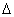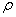## Electrical CurrentElectric current is the flow of charge, much like water currents are the flow of water molecules. Water molecules tend to flow from areas of high gravitational potential energy to low gravitational potential energy. Electric currents flow from high electric potential to low electric potential. And the greater the difference between the high and low potential, the more current that flows!

In a majority of electric currents, the moving charges are negative electrons. However, due to historical reasons dating back to Ben Franklin, we say that conventional current flows in the direction positive charges would move. Although inconvenient, it’s fairly easy to keep straight if you just remember that the actual moving charges, the electrons, flow in a direction opposite that of the electric current. With this in mind, we can state that positive current flows from high potential to low potential, even though the charge carriers (electrons) actually flow from low to high potential.

Electric current (I) is measured in amperes (A), or amps, and can be calculated by finding the total amount of charge (q), in Coulombs, which passes a specific point in a given time (t). Electric current can therefore be calculated as:Question: A charge of 30 Coulombs passes through a 24-ohm resistor in 6.0 seconds. What is the current through the resistor?

Answer:## Introduction to Current Electricity##### Flow of ChargeElectric current is the flow of charge, much like water currents are the flow of water molecules. Water molecules tend to flow from areas of high gravitational potential energy to low gravitational potential energy. Electric currents flow from high electric potential to low electric potential. And the greater the difference between the high and low potential, the more current that flows!

In a majority of electric currents, the moving charges are negative electrons. However, due to historical reasons dating back to Ben Franklin, we say that conventional current flows in the direction positive charges would move. Although inconvenient, it’s fairly easy to keep straight if you just remember that the actual moving charges, the electrons, flow in a direction opposite that of the electric current. With this in mind, we can state that positive current flows from high potential to low potential, even though the charge carriers (electrons) actually flow from low to high potential.

Electric current (I) is measured in amperes (A), or amps, and can be calculated by finding the total amount of charge (q), in Coulombs, which passes a specific point in a given time (t). Electric current can therefore be calculated as:Question: A charge of 30 Coulombs passes through a 24-ohm resistor in 6.0 seconds. What is the current through the resistor?

Answer:##### Resistance

Electrical charges can move easily in some materials (conductors) and less freely in others (insulators), as we learned previously. We describe a material’s ability to conduct electric charge as conductivity. Good conductors have high conductivities. In similar fashion, we describe a material’s ability to resist the movement of electric charge using resistivity, symbolized with the Greek letter rho (). Resistivity is measured in ohm-meters, which are represented by the Greek letter omega multiplied by meters (•m). Both conductivity and resistivity are properties of a material.

Ball Lightning Sample: